Function Repository Resource:

# ButcherTreeCount

Get a list of the number of Butcher trees through a given order

Contributed by: Wolfram Research
 ResourceFunction["ButcherTreeCount"][p] gives a list of the number of trees through order p.

## Examples

### Basic Examples (1)

Determine the number of trees at each order through order 10:

 In:=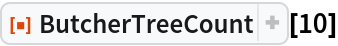Out=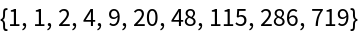### Scope (1)

The result of ButcherTreeCount can be used to calculate the total number of trees required at each order:

 In:=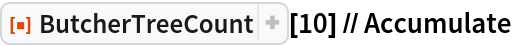Out=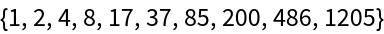### Properties and Relations (1)

The result is equivalent to evaluating the length of each output of the resource function RungeKuttaOrderConditions at each level, but the calculation is more efficient:

 In:=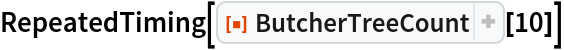Out=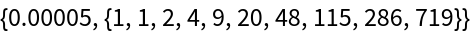In:=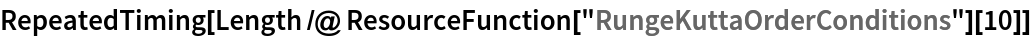Out=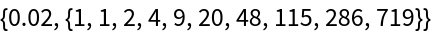## Version History

• 1.0.0 – 03 September 2019